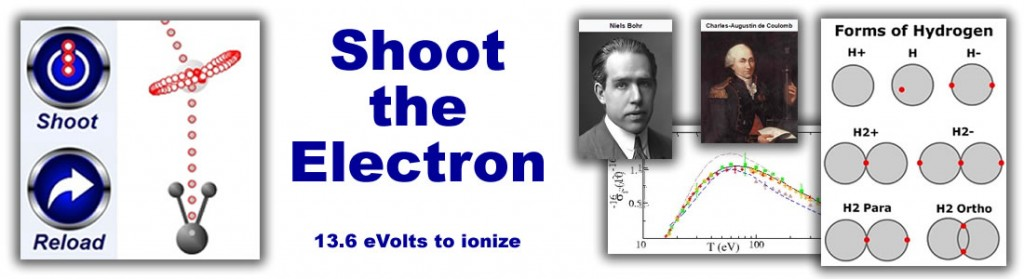# Electrons, Protons and the Coulomb ForceBefore modelling protons and electrons under the coulomb force, a quick note about time scales and distance scales. Distances on the atomic scale for protons and atoms are often measured in picometers (10‾¹²), where the Bohr radius is 53pm. For electrons, distances can go as small as the Lorentz radius of 3 femtometers (10‾¹⁵). For tracking electrons, attoseconds (10‾¹⁸) is a common time scale. Protons do not appear to move at all at this time scale. Protons and other nucleons, being thousands of times heavier then the electron, sit in one spot while the electrons buzz around.

Outside the Bohr radius of 53pm, Coulomb’s law does a pretty good job of modelling electrons and protons. Once the distances get closer than this, things start to break down and you have to start to think about how to model the quantum mechanical effects.

Consider an electron falling towards a proton. Using Coulomb’s law, it will cross the Bohr radius at a speed of about 2.2 picometers per attosecond. For reference, the speed of light is 300 picometers per attosecond. The speed of the electron is related to its energy (measured in evolts) by the formula E=½mv². This means an electron with 10eV energy will be moving at 1.9 picos/attosec. An electron at 100eV will travel at 6.0 picos/attosec. An electron must have an energy of at least 13.6 eVolts to escape from the proton. This is the ionization energy of Hydrogen, or put another way, the amount of energy required to pull the electron away from the proton.

Under Coulomb’s law, the Bohr radius is the distance where an electron with less then 13.6eV energy cannot escape the proton. But what is more important in terms of a model, no matter how close the electron gets to the proton, it never takes more then 13.6eV to pull it away. This is clearly a breakdown of Coulomb’s law (only the first of many as we will see) and something must be introduced to the animation to reflect this breakdown.

#### Newton’s Shell Theorem

One model that works pretty well, derives a world made up of tiny electrons and large empty proton shells. Using Newton’s shell theorem as support, a world is modelled where the electrons do not feel an attraction to the proton once inside the proton shell. Under these forces, the electron does not spiral into the proton while emitting radiation since it feels no force from the proton inside the shell. The model traps electrons between energies from 0 eVolts to 13.6 eVolts. Anything larger then 13.6 eVolts and the electron will fly off into space.

In programming terms the force on the electron, Force[E₁], is determined by K, the Coulomb constant; PCharge, the charge of the proton; and D, the distance between the electron and proton.

if D>53pm then Force[E₁] = K*PCharge/D²
if D<=53pm then Force[E₁] = 0

We can expand this model and have a look at the kind of orbit that two electrons and one proton produce. The animation on the right shows two electrons bouncing around with 2.0eV of energy between them.

We can also expand this model with multiple protons. An electron inside the Bohr radius of a proton becomes trapped in this model and cannot get out. It can easily become trapped within the Bohr radius of a second proton, effectively bonding the two protons together.